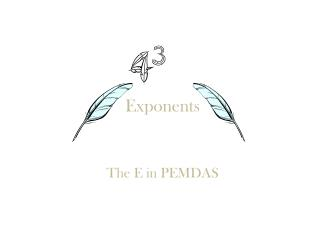DownloadDownload PresentationExponents

# Exponents

Télécharger la présentation## Exponents

- - - - - - - - - - - - - - - - - - - - - - - - - - - E N D - - - - - - - - - - - - - - - - - - - - - - - - - - -
##### Presentation Transcript

1. Exponents The E in PEMDAS

2. Exponents • Exponents are used everyday you may just not know it. 32 is an exponent but which number is verified as an exponent? The two! So in the numbers below what are the exponents? 42 35 83 Exponents are actually very close to multiplication problems. 42 is the same as 4x4. You may wonder why is there a 2 not a 4 as the exponent. Well the two says that it’s two fours multiplying each other which says 4x4.

3. Squaring • Squaring may seem weird, but is actually is the same as using exponents. Only when there is a 2 is it called squaring. 32, 42, and 82 are all used when squaring. In area squaring is used very often. Three inches squared is an example.

4. PEMDAS properties • PEMDAS is the order of operations. The order of operations is Parentheses, exponents, multiplication, division, addition, and subtraction. PEMDAS shows that parentheses are 1st and exponents are 2nd. If parentheses are not there exponents are to be done next. Here is an example. 33x2-6= 27x2-6= 54-6=48 final answer is 48

5. Exponent game • To win, find out which answer's digits sum up to seven. That means that if an answer were 92 the question would be what numbers digits sum up to eleven . (92) 9+2=11 Answer the questionright to get the gold, but if you get it wrong the agency will find you. You must answer them all! • 33 • 53 • 82 • 52 Did that secret agency find you?

6. Exponent game • Digits sum to 12, Answer all 43 72 92 22 Thanks for playing!

7. BYE,BYE!